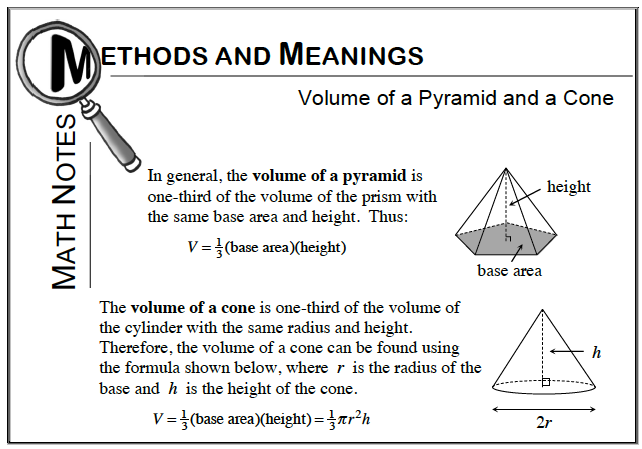Home > CC3 > Chapter Ch10 > Lesson 10.1.4 > Problem10-57

10-57.
1. Find the volume of each shape below. Homework Help ✎

1. Rectangle-based prism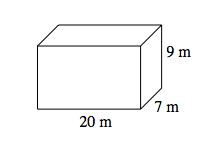2. Rectangle-based pyramid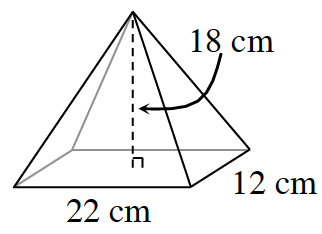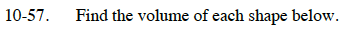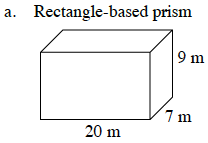The volume of a rectangle-based prism is:
V = (base area) (height)

Substitute the values given.
V = [(20m)(7m)] [9m]

Evaluate.
V = [140 m²][9m]

1260 m³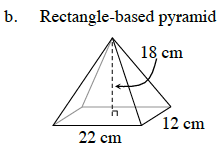Refer to the Math Notes box below for the equation to find the volume of a pyramid. Then follow the steps from part (a).